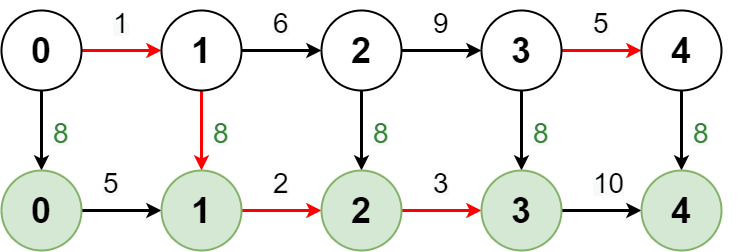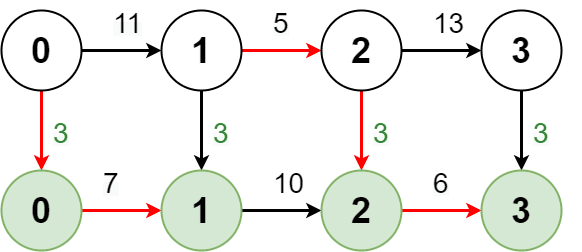##### Welcome to Subscribe On Youtube

Formatted question description: https://leetcode.ca/all/2361.html

# 2361. Minimum Costs Using the Train Line

## Description

A train line going through a city has two routes, the regular route and the express route. Both routes go through the same n + 1 stops labeled from 0 to n. Initially, you start on the regular route at stop 0.

You are given two 1-indexed integer arrays regular and express, both of length n. regular[i] describes the cost it takes to go from stop i - 1 to stop i using the regular route, and express[i] describes the cost it takes to go from stop i - 1 to stop i using the express route.

You are also given an integer expressCost which represents the cost to transfer from the regular route to the express route.

Note that:

• There is no cost to transfer from the express route back to the regular route.
• You pay expressCost every time you transfer from the regular route to the express route.
• There is no extra cost to stay on the express route.

Return a 1-indexed array costs of length n, where costs[i] is the minimum cost to reach stop i from stop 0.

Note that a stop can be counted as reached from either route.

Example 1:Input: regular = [1,6,9,5], express = [5,2,3,10], expressCost = 8
Output: [1,7,14,19]
Explanation: The diagram above shows how to reach stop 4 from stop 0 with minimum cost.
- Take the regular route from stop 0 to stop 1, costing 1.
- Take the express route from stop 1 to stop 2, costing 8 + 2 = 10.
- Take the express route from stop 2 to stop 3, costing 3.
- Take the regular route from stop 3 to stop 4, costing 5.
The total cost is 1 + 10 + 3 + 5 = 19.
Note that a different route could be taken to reach the other stops with minimum cost.


Example 2:Input: regular = [11,5,13], express = [7,10,6], expressCost = 3
Output: [10,15,24]
Explanation: The diagram above shows how to reach stop 3 from stop 0 with minimum cost.
- Take the express route from stop 0 to stop 1, costing 3 + 7 = 10.
- Take the regular route from stop 1 to stop 2, costing 5.
- Take the express route from stop 2 to stop 3, costing 3 + 6 = 9.
The total cost is 10 + 5 + 9 = 24.
Note that the expressCost is paid again to transfer back to the express route.


Constraints:

• n == regular.length == express.length
• 1 <= n <= 105
• 1 <= regular[i], express[i], expressCost <= 105

## Solutions

• class Solution {
public long[] minimumCosts(int[] regular, int[] express, int expressCost) {
int n = regular.length;
long f = 0;
long g = 1 << 30;
long[] cost = new long[n];
for (int i = 0; i < n; ++i) {
int a = regular[i];
int b = express[i];
long ff = Math.min(f + a, g + a);
long gg = Math.min(f + expressCost + b, g + b);
f = ff;
g = gg;
cost[i] = Math.min(f, g);
}
return cost;
}
}

• class Solution {
public:
vector<long long> minimumCosts(vector<int>& regular, vector<int>& express, int expressCost) {
int n = regular.size();
long long f = 0;
long long g = 1 << 30;
vector<long long> cost(n);
for (int i = 0; i < n; ++i) {
int a = regular[i];
int b = express[i];
long long ff = min(f + a, g + a);
long long gg = min(f + expressCost + b, g + b);
f = ff;
g = gg;
cost[i] = min(f, g);
}
return cost;
}
};

• class Solution:
def minimumCosts(
self, regular: List[int], express: List[int], expressCost: int
) -> List[int]:
n = len(regular)
f, g = 0, inf
cost =  * n
for i, (a, b) in enumerate(zip(regular, express), 1):
ff = min(f + a, g + a)
gg = min(f + expressCost + b, g + b)
f, g = ff, gg
cost[i - 1] = min(f, g)
return cost


• func minimumCosts(regular []int, express []int, expressCost int) []int64 {
f, g := 0, 1<<30
cost := make([]int64, len(regular))
for i, a := range regular {
b := express[i]
ff := min(f+a, g+a)
gg := min(f+expressCost+b, g+b)
f, g = ff, gg
cost[i] = int64(min(f, g))
}
return cost
}

func min(a, b int) int {
if a < b {
return a
}
return b
}

• function minimumCosts(
regular: number[],
express: number[],
expressCost: number,
): number[] {
const n = regular.length;
let f = 0;
let g = 1 << 30;
const cost: number[] = new Array(n).fill(0);
for (let i = 0; i < n; ++i) {
const [a, b] = [regular[i], express[i]];
const ff = Math.min(f + a, g + a);
const gg = Math.min(f + expressCost + b, g + b);
[f, g] = [ff, gg];
cost[i] = Math.min(f, g);
}
return cost;
}